# Maths Worksheet for Class 3Maths Worksheet for Class 3 is a perfect amalgamation of fun and learning for young minds to get a good grasp of major mathematical operations. Depending entirely on the school for your kid’s learning is not a great option in this highly competitive world. Whatever the kids learn at school must be revised at home. Making them go through the theory of the concept, again and again, will result in their disinterest in the subject.

That’s why we have worksheets. Worksheets are proven to be one of the best resources to sharpen kids’ concepts of various subjects. Worksheets don’t contain theory. They just refine the topics through a lot of practice questions and puzzles.

To help you assist your kid in practising at home, we are providing you with the following Maths Worksheet for Class 3. You can also download these Class 3 Maths Worksheet pdf through the link below.

## 1. Division Sums for Class 3

Here is a set of CBSE Class 3 Maths Division Worksheets. The Division worksheet for Class 3 kids will certainly engage your kid in practising the division concepts in a fun way. You can download these worksheets containing Division Questions for Class 3, through the given links.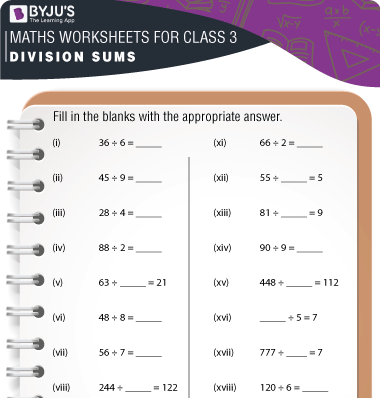##### Division Sums for Class 3 Worksheet-1

Explore practice questions on division sums for class 3. Here, the student has to fill in the blank with appropriate numbers.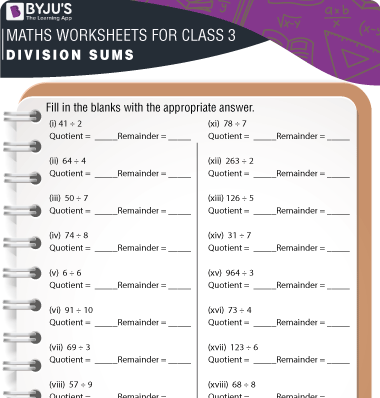##### Division Sums for Class 3 Worksheet-2

In this worksheet, the student needs to fill in the blanks with the quotients and remainders for the given sums.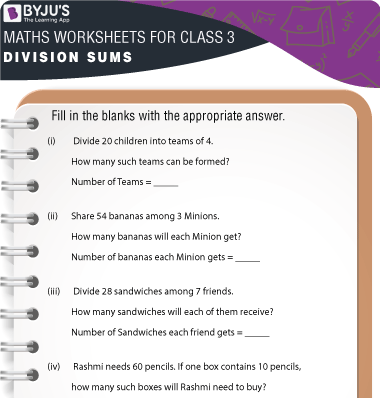##### Division Sums for Class 3 Worksheet-3

Here we have word problems based on division. No practice is complete without solving some intricate word problems based on that operation.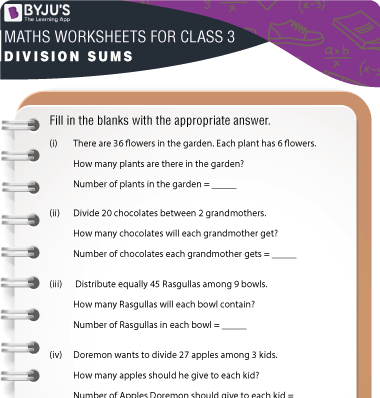##### Division Sums for Class 3 Worksheet-4

Find here brain-tickling division sums word problems for young minds. Explore how well you are able to solve these questions.

## 2. Multiplication Sums for Class 3

We provide you with Multiplication Worksheet for Class 3 which contains several multiplication word problems. It also has Multiply sums for Class 3 kids. You can download these Multiplication Questions through the links in PDF Format and let your kid enjoy solving interesting questions on multiplication.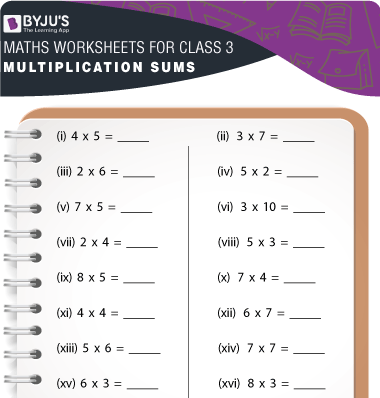##### Multiplication sums for class 3 Worksheet-1

Get here simple multiplication sums for Class 3 kids. The students need to fill the blanks with appropriate answers.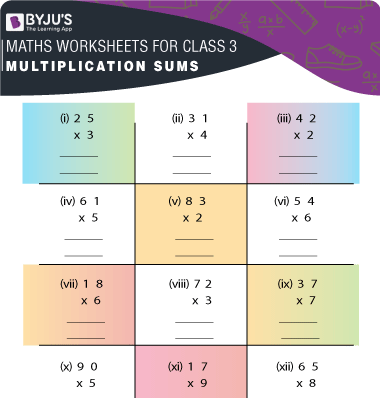##### Multiplication sums for class 3 Worksheet-2

A colourful representation of the traditional multiplication layout.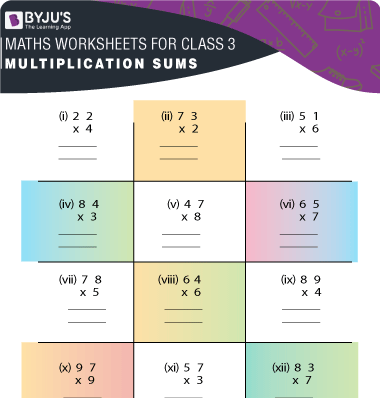##### Multiplication sums for class 3 Worksheet-1

Here, the students have to write the correct answers to the multiplication sums provided on the worksheet.

## 3. Subtraction Sums for Class 3

Questions on Subtraction for Class 3 is focussed towards giving kids a better idea about subtraction of two numbers comprising different number of digits. To help you assist your kid better, we have Subtraction Worksheet for Class 3, which you can download in PDF format.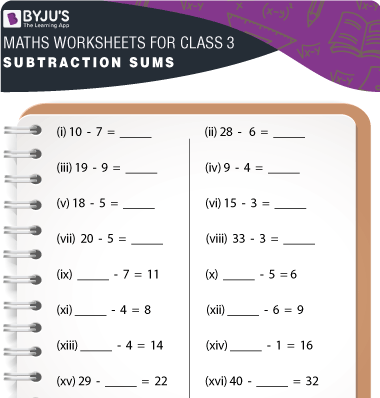##### Subtraction Sums for Class 3 Worksheet-1

Explore this worksheet on Subtraction sums for Class 3 students. Here, students need to fill the blanks with appropriate answers.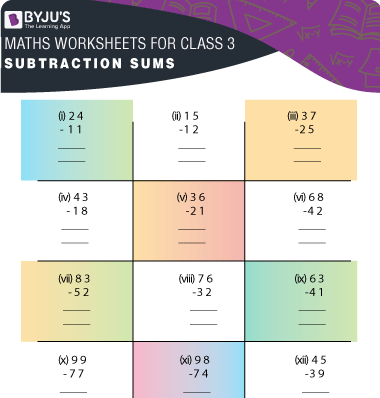##### Subtraction Sums for Class 3 Worksheet-2

This worksheet on subtraction sums for Class 3 is designed in the traditional structure of subtraction and has double-digit subtraction.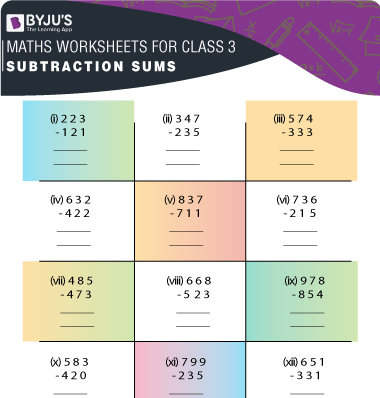##### Subtraction Sums for Class 3 Worksheet-3

A worksheet on subtraction sums for Class 3, this has challenging three-digit subtraction sums for students.

## 4. Addition Sums for Class 3

Addition is one of the major arithmetic operations that is aimed at in the primary classes. A good understanding of basic mathematical operations is very crucial for having a good grasp on tougher problems, which students come across in their later years of education. Here, we present you a set of Addition Worksheet for Class 3 students. You can download them in PDF format, through the links.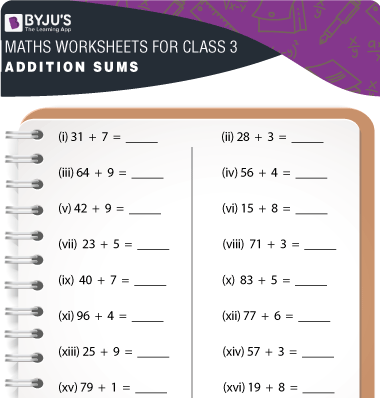##### Addition Sums for Class 3 Worksheet-1

A worksheet with questions on Addition sums for Class 3, this has simple addition questions where the students are supposed to fill the blanks with appropriate answers.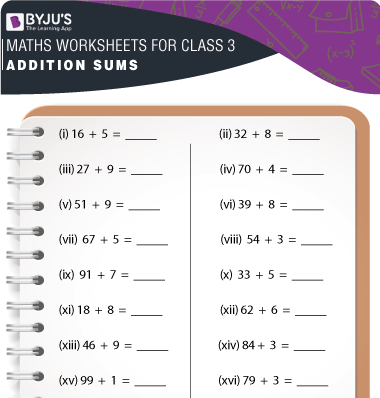##### Addition Sums for Class 3 Worksheet-2

Explore this simple worksheet on Addition sums for Class 3 kids. The students need to fill the blanks with proper answers.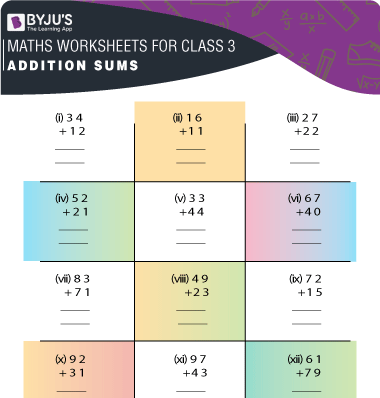## 5. Word Problems for Class 3

Word problems are intriguing questions to solve for students of all ages. Kids are introduced to simple word problems in their primary classes. We bring to you a set of Maths Word Problems for Class 3 students in order to get them ready for complex word problems.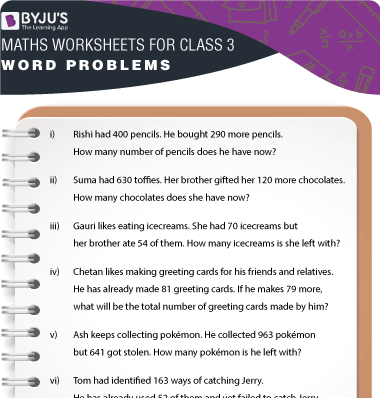##### Word Problems for Class 3 Worksheet-1

One of the most challenging types of questions are word problems. Explore this worksheet on addition and subtraction based word problems for Class 3 kids.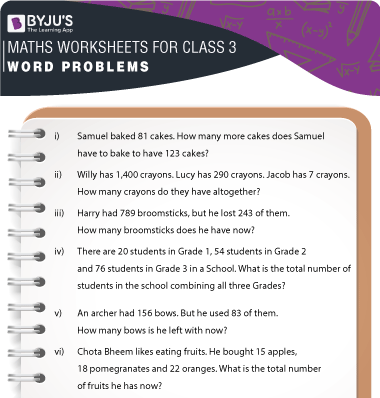##### Word Problems for Class 3 Worksheet-2

This worksheet on word problems brain-tickles Class 3 students by its questions based on addition and subtraction.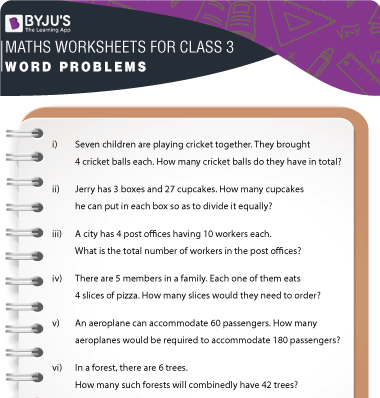##### Word Problems for Class 3 Worksheet-3

Multiplication and Division are a little tough for kids. Explore this worksheet with word problems based on multiplication and division for Class 3 students.

These worksheets contain interesting puzzles and exciting Maths sums for Class 3. These worksheets also carry questions on mental Maths. They have been designed meticulously by our subject-matter experts. They have crafted the worksheets keeping in mind the learning capacity of kids and their short attention span.

The questions have been structured similar to the ones in the CBSE Class 3 maths worksheets. These Class 3 questions are taken to build a better understanding of clocks and calendars, place values and face values, predecessor and successor, basics of geometry, etc. You can get these CBSE Class 3 Maths question PDFs here. Solving these exercises will surely help your kid to excel in Mathematics right from the beginning of his/her learning.

The curriculum of Class 3 also comprises the story sums for Class 3 kids. Story sums are indirect questions which kids have to solve by deciding what operation must be performed to find the solution to the sum. The operation might be any of the arithmetic operations – addition, subtraction, multiplication or division. Kids need to read the question thoroughly and decide as to which mathematical operation will produce the desired results.